Practice
Resources
Contests
Online IDE
New
Free Mock
Scaler
Practice
Improve your coding skills with our resources
Contests
Compete in popular contests with top coders
Scaler
Explore Offerings by SCALER## Welcome to Interviewbit, help us create the best experience for you!

Currently, You are a:College/University *
Enter the name of your college
Branch *
Year of completion *College/University *
Enter the name of your college
Branch *
Year of completion *Current Company *
Enter company name
Experience *## You're all set!Full name *
Email *

By creating an account, I acknowledge that I have read and agree to InterviewBit’s Terms and Privacy Policy .## Welcome back!

Email *Go to Problems

Be a Code Ninja!

# Selection Sort

Selection sort is a simple comparison-based sorting algorithm. It is in-place and needs no extra memory.

The idea behind this algorithm is pretty simple. We divide the array into two parts: sorted and unsorted. The left part is sorted subarray and the right part is unsorted subarray. Initially, sorted subarray is empty and unsorted array is the complete given array.

We perform the steps given below until the unsorted subarray becomes empty:

1. Pick the minimum element from the unsorted subarray.
2. Swap it with the leftmost element of the unsorted subarray.
3. Now the leftmost element of unsorted subarray becomes a part (rightmost) of sorted subarray and will not be a part of unsorted subarray.

## A selection sort works as follows:Part of unsorted arrayPart of sorted arrayLeftmost element in unsorted arrayMinimum element in unsorted array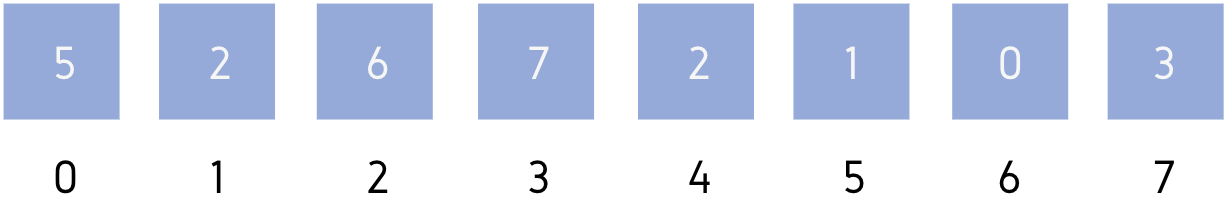This is our initial array A = [5, 2, 6, 7, 2, 1, 0, 3]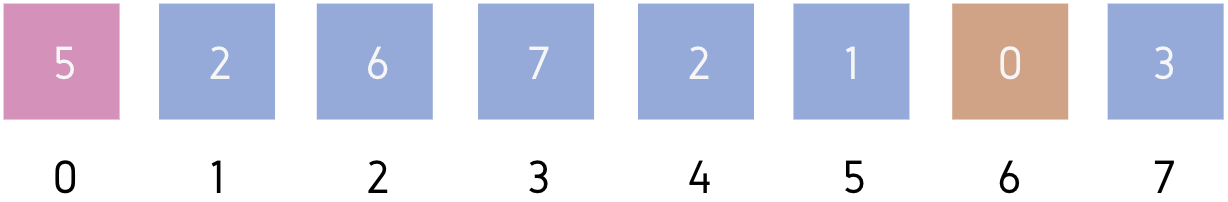Leftmost element of unsorted part = A

Minimum element of unsorted part = A

We will swap A and A then, make A part of sorted subarray.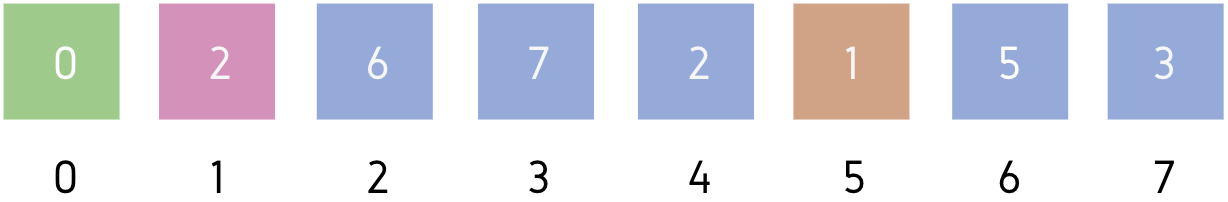Leftmost element of unsorted part = A

Minimum element of unsorted part = A

We will swap A and A then, make A part of sorted subarray.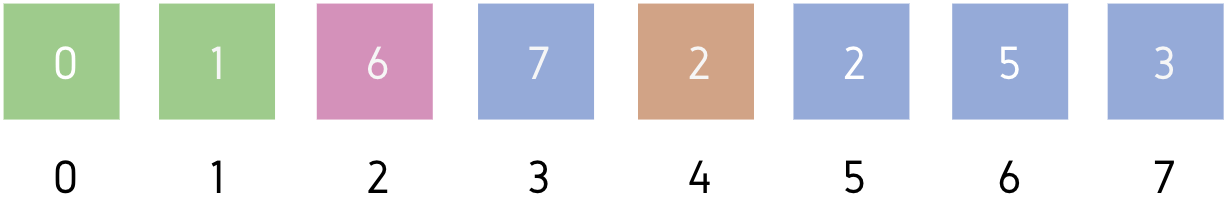Leftmost element of unsorted part = A

Minimum element of unsorted part = A

We will swap A and A then, make A part of sorted subarray.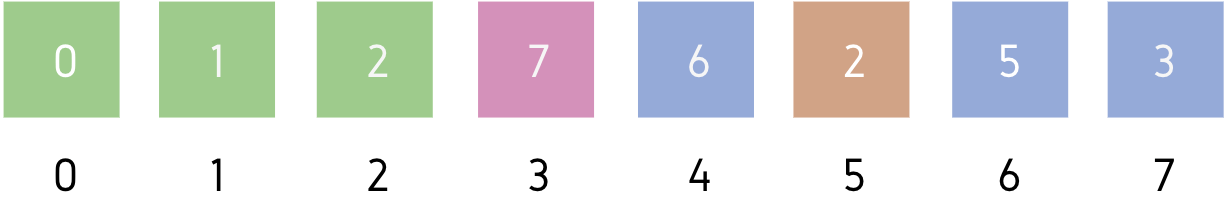Leftmost element of unsorted part = A

Minimum element of unsorted part = A

We will swap A and A then, make A part of sorted subarray.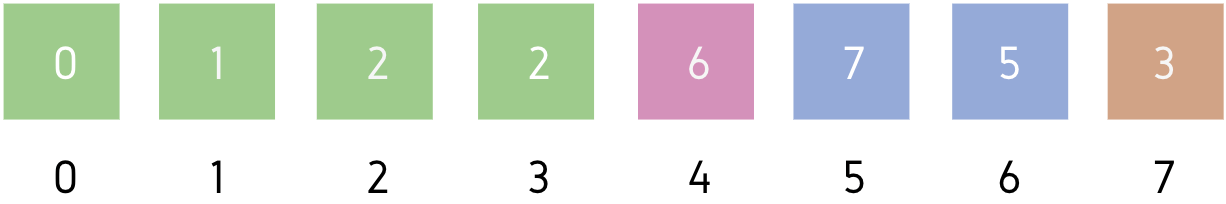Leftmost element of unsorted part = A

Minimum element of unsorted part = A

We will swap A and A then, make A part of sorted subarray.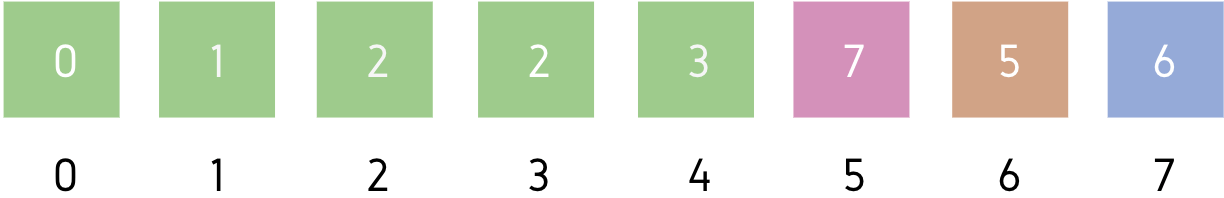Leftmost element of unsorted part = A

Minimum element of unsorted part = A

We will swap A and A then, make A part of sorted subarray.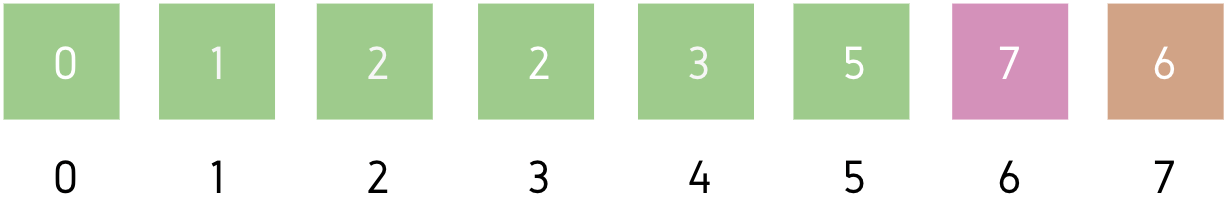Leftmost element of unsorted part = A

Minimum element of unsorted part = A

We will swap A and A then, make A part of sorted subarray.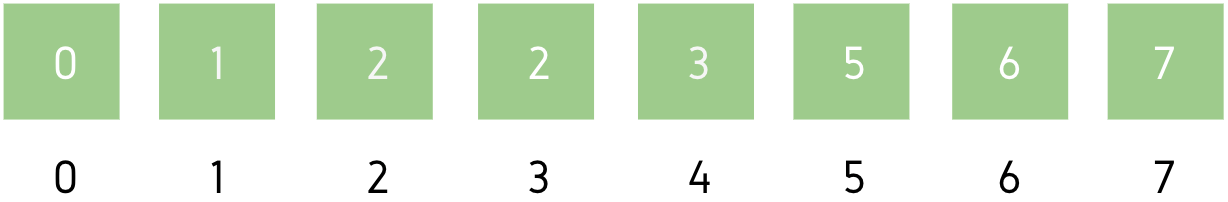This is the final sorted array.

### Pseudocode

FindMinIndex:

``````FindMinIndex(Arr[], start, end)
min_index = start

FOR i from (start + 1) to end:
IF Arr[i] < Arr[min_index]:
min_index = i
END of IF
END of FOR

Return min_index``````

Suppose, there are ‘n’ elements in the array. Therefore, at worst case, there can be n iterations in FindMinIndex() for start = 1 and end = n. We did not take any auxiliary space.

Therefore,

Time complexity: O(n)

Space complexity: O(1)

Selection Sort:

``````SelectionSort(Arr[], arr_size):
FOR i from 1 to arr_size:
min_index = FindMinIndex(Arr, i, arr_size)

IF i != min_index:
swap(Arr[i], Arr[min_index])
END of IF
END of FOR``````

Suppose, there are ‘n’ elements in the array. Therefore, at worst case, there can be n iterations in FindMinIndex() for start = 1 and end = n. No auxiliary space used.

Total iterations = (n – 1) + (n – 2) + . . . + 1 = (n * (n – 1)) / 2 = (n2 – n) / 2

Therefore,

Time complexity: O(n2)

Space complexity: O(1)

### Implementation:

Following are C, C++, Java and Python implementations of Selection Sort.

``````Selection sort program in C:

#include <stdio.h>

void swap(int *A, int i, int j) {
int temp = A[i];
A[i] = A[j];
A[j] = temp;
}

int findMinIndex(int *A, int start, int end) {
int min_index = start;

++start;

while(start < end) {
if(A[start] < A[min_index])
min_index = start;

++start;
}

return min_index;
}

void selectionSort(int *A, int n) {
for(int i = 0; i < n - 1; ++i) {
int min_index = findMinIndex(A, i, n);

if(i != min_index)
swap(A, i, min_index);
}
}

int main() {
int A[] = {5, 2, 6, 7, 2, 1, 0, 3}, n = 8;

selectionSort(A, n);

for(int i = 0; i < n; ++i)
printf("%d ", A[i]);
return 0;
}``````
``````Selection sort program in C++:

#include <iostream>
#include <vector>

using namespace std;

int findMinIndex(vector<int> &A, int start) {
int min_index = start;

++start;

while(start < (int)A.size()) {
if(A[start] < A[min_index])
min_index = start;

++start;
}

return min_index;
}

void selectionSort(vector<int> &A) {
for(int i = 0; i < (int)A.size(); ++i) {
int min_index = findMinIndex(A, i);

if(i != min_index)
swap(A[i], A[min_index]);
}
}

int main() {
vector<int> A = {5, 2, 6, 7, 2, 1, 0, 3};

selectionSort(A);

for(int num : A)
cout << num << ' ';

return 0;
} ``````
``````Selection Sort Program in Java

class SelectionSort {
void swap(int A[], int i, int j) {
int temp = A[i];
A[i] = A[j];
A[j] = temp;
}

int findMinIndex(int A[], int start) {
int min_index = start;

++start;

while(start < A.length) {
if(A[start] < A[min_index])
min_index = start;

++start;
}

return min_index;
}

void selectionSort(int A[]) {
for(int i = 0; i < A.length; ++i) {
int min_index = findMinIndex(A, i);

if(i != min_index)
swap(A, i, min_index);
}
}

public static void main(String[] args) {
int A[] = {5, 2, 6, 7, 2, 1, 0, 3};

selectionSort(A);

for(int num : A)
System.out.print(num + " ");

return 0;
}
}``````
``````Selection Sort Program in Python

def findMinIndex(A, start):
min_index = start

start += 1

while start < len(A):
if A[start] < A[min_index]:
min_index = start

start += 1

return min_index

def selectionSort(A):
i = 0

while i < len(A):
min_index = findMinIndex(A, i)

if i != min_index:
A[i], A[min_index] = A[min_index], A[i]

i += 1

A = [5, 2, 6, 7, 2, 1, 0, 3]

selectionSort(A)

for num in A:
print(num, end=' ')``````

## Serious about Learning Programming ?

Learn this and a lot more with Scaler Academy's industry vetted curriculum which covers Data Structures & Algorithms in depth.
Primers
Examples

## Arrays Problems

Array math
Value ranges
Problem Score Companies Time Status
Max Min 150
17:31
Merge Intervals 225 78:57
Merge Overlapping Intervals 225 48:24
Simulation array
Problem Score Companies Time Status
Perfect Peak of Array 200 48:49
Kth Row of Pascal's Triangle 225
28:32
Spiral Order Matrix II 225 48:40
Pascal Triangle 225 26:46
Anti Diagonals 225 41:46
Bucketing
Problem Score Companies Time Status
Triplets with Sum between given range 200
76:05
Balance Array 200 61:34
Find Duplicate in Array 450 40:13
Maximum Consecutive Gap 450 58:46
Arrangement
Problem Score Companies Time Status
Sort array with squares! 200 31:18
Largest Number 225 70:26
Rotate Matrix 300 60:26
Next Permutation 300 63:13
Find Permutation 300 56:00
Sorting
Problem Score Companies Time Status
Noble Integer 200 43:30
Wave Array 225 22:08
Hotel Bookings Possible 225 66:06
Max Distance 250 68:14
Maximum Unsorted Subarray 250 68:52
Space recycle
Problem Score Companies Time Status
Set Matrix Zeros 300 48:04
First Missing Integer 300 64:38
Maximum Sum Square SubMatrix 300 56:27
Missing / repeated number
Problem Score Companies Time Status
First Missing Integer 300 64:38
Repeat and Missing Number Array 350 63:55
N/3 Repeat Number 600
68:22

Problem Score Companies Time Status
Reorder Data in Log Files 200 42:12
Move Zeroes 200 24:32
Make equal elements Array 200 28:24
Segregate 0s and 1s in an array 200 14:16
Array Sum 200 30:46
Set Intersection 200 60:40
Occurence of Each Number 200 22:33
Greater of Lesser 200 12:09
Spiral Matrix 200 30:25
Product of All 200 29:50
Chips Factory 200 17:44
Greater than All 150 17:09
Pythagorean Triplets 200 22:34
Diagonal Flip 200 16:57
Positive Negative 100 9:33Free Mock Assessment
Help us know you better for the best experience
Current Employer *
Enter company name